Next: Combinatorial analysis Up: Probability theory Previous: Combining probabilities

## The two-state system

The simplest non-trivial system which we can investigate using probability theory is one for which there are only two possible outcomes. There would obviously be little point in investigating a one outcome system. Let us suppose that there are two possible outcomes to an observation made on some system. Let us denote these outcomes 1 and 2, and let their probabilities of occurrence be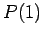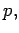(9)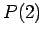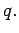(10)

It follows immediately from the normalization condition (5) that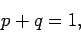(11)

so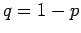. The best known example of a two-state system is a tossed coin. The two outcomes are heads'' and tails,'' each with equal probabilities. So,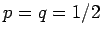for this system.

Suppose that we makestatistically independent observations of. Let us determine the probability of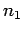occurrences of the outcome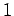andoccurrences of the outcome 2, with no regard to the order of these occurrences. Denote this probability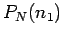. This type of calculation crops up again and again in probability theory. For instance, we might want to know the probability of getting nine heads'' and only one tails'' in an experiment where a coin is tossed ten times, or where ten coins are tossed simultaneously.

Consider a simple case in which there are only three observations. Let us try to evaluate the probability of two occurrences of the outcome 1 and one occurrence of the outcome 2. There are three different ways of getting this result. We could get the outcome 1 on the first two observations and the outcome 2 on the third. Or, we could get the outcome 2 on the first observation and the outcome 1 on the latter two observations. Or, we could get the outcome 1 on the first and last observations and the outcome 2 on the middle observation. Writing this symbolically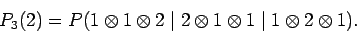(12)

This formula looks a bit scary, but all we have done here is to write out symbolically what was just said in words. Where we said and'' we have written the symbolic operator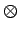, and where we said or'' we have written the symbolic operator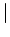. This symbolic representation is helpful because of the two basic rules for combining probabilities which we derived earlier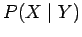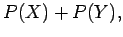(13)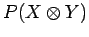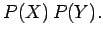(14)

The straightforward application of these rules gives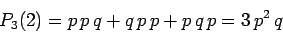(15)

in the case under consideration.

The probability of obtainingoccurrences of the outcomeinobservations is given by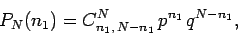(16)

where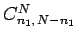is the number of ways of arranging two distinct sets ofandindistinguishable objects. Hopefully, that this is, at least, plausible from the example we just discussed. There, the probability of getting two occurrences of the outcome 1 and one occurrence of the outcome 2 was obtained by writing out all of the possible arrangements of two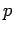s (the probability of outcome 1) and one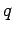(the probability of outcome 2), and then added them all together.Next: Combinatorial analysis Up: Probability theory Previous: Combining probabilities
Richard Fitzpatrick 2006-02-02